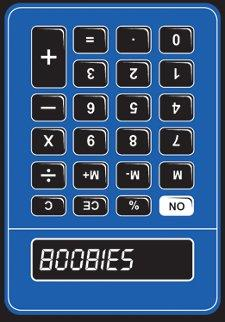# MATH TIME!

Discussion in 'User Topics' started by Mr.Lame, Jan 9, 2018.

1. @MarkFL give me the average percentage out of these:

63%
25%
33.3%
62%
45.8%
25%

And then the average out of these percentages:

53.8%
35.3%
27.8%
50.7%
56.5%
66.7%

Cba to figure it out myself, I'm tutoring chess and these are best move percentages across 6 of their games. Want to find out what the average was.

2. @Mr.Lame

3. Nvm remembered Mr. Wilkinson's year 5 class on how to figure out the mean

•Funny x 1
4. I was never a maths person, leave me alone!

•Funny x 1
5. In words, that formula tells you that to find the arithmetic mean (average - denoted by x-bar), add up the numbers, then divide the resulting sum by the number of numbers (which I assume you did).•Like x 1
6. Oh, I thought you were mocking me by posting some complex formula.

7. I will admit that I was trying to be a little "math nerdy" but not mockery was intended.•Like x 2
8. I bet the equation means "**** you" in maths language

•Funny x 2
9. No, I assure you it simply states how to compute the mean of a set of numbers. Mathematics is meant to be useful, not derisive.10.•Funny x 3